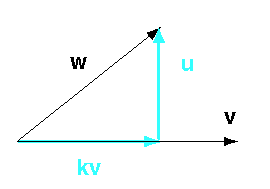Yes, unless the two vectors are parallel.

# Two Vectors Define a PlaneYou may recall from high school geometry that two lines (not colinear) define a plane. This is the same thing. Most of the examples in this chapter start with two 2D vectors. However, the results are valid for two 3D vectors because the two 3D vectors define a plane.

The figure shows a vector w and a vector v. For convenience in visualization, their tails start from the same point (but, of course, vectors have no position).

Also for convenience, the vector v is horizontal; but it could be at any orientation.

### QUESTION 3:

Does the scaled vector kv (where k is a scalar) have the same orientation as v?